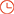﻿ 广州市自来水有限公司2022～2024年度二次供水加压设备招标项目(第三包)招标公告_中国电力招标网
当前位置：首页 > 招标采购 > 工程招标 > 招标信息

 所属地区 广东 投标截止时间 *** 登录后查看 资质要求 注册 或者 登录 后查看 开标时间设置开标提醒 项目进展 购买会员服务 代购标书 中标监测

*受广州市自来水有限公司（以下简称招标人）的委托，就以下采购项目进行公开招标，欢迎符合资格条件的投标人投标。
1. 招标项目简介
1.1 项目名称
1.1.1. 招标项目名称：广州市自来水有限公司2022～2024年度二次供水加压设备公开招标项目
1.1.2 工程项目名称：
（1） 广州市自来水有限公司2022～2024年度二次供水加压设备公开招标项目（第一包）
（2） 广州市自来水有限公司2022～2024年度二次供水加压设备公开招标项目（第二包）
（3） 广州市自来水有限公司2022～2024年度二次供水加压设备公开招标项目（第三包）
1.2. 项目编号：GZCZ621DL1000467
1.3. 本招标项目采购预算（或最高投标限价）和材料清单：

 序号 设备、材料名称/水泵性能参数 电压等级/规格 单位 数量 备注 1 工频二次供水加压设备 Q=2m3/h、H=40m、P=0.75kW、220V 套 1 2 Q=2m3/h、H=40m、P=0.75kW、380V 套 1 3 Q=4m3/h、H=40m、P=1.1kW、220V 套 4 4 Q=4m3/h、H=40m、P=1.1kW、380V 套 1 5 Q=6m3/h、H=40m、P=1.5kW、220V 套 4 6 Q=6m3/h、H=40m、P=1.5kW、380V 套 1 7 Q=10m3/h、H=40m、P=2.2kW、220V 套 1 8 Q=10m3/h、H=40m、P=2.2kW、380V 套 1 9 Q=12m3/h、H=40m、P=2.2kW、220V 套 1 10 Q=12m3/h、H=40m、P=2.2kW、380V 套 1 11 Q=4m3/h、H=48m、P=1.5kW、220V 套 4 12 Q=4m3/h、H=48m、P=1.5kW、380V 套 1 13 Q=6m3/h、H=48m、P=2.2kW、220V 套 4 14 Q=6m3/h、H=48m、P=2.2kW、380V 套 1 15 Q=8m3/h、H=48m、P=2.2kW、220V 套 3 16 Q=8m3/h、H=48m、P=2.2kW、380V 套 1 17 Q=10m3/h、H=48m、P=3kW、380V 套 1 18 Q=12m3/h、H=48m、P=3kW、380V 套 1 19 Q=15m3/h、H=48m、P=4kW、380V 套 1 20 Q=24m3/h、H=48m、P=5.5kW、380V 套 1 21 Q=24m3/h、H=78m、P=11kW、380V 套 1 22 变频二次供水加压设备 Q=2m3/h、H=40m、P=0.75kW、220V 套 1 23 Q=2m3/h、H=40m、P=0.75kW、380V 套 1 24 Q=4m3/h、H=40m、P=1.1kW、220V 套 4 25 Q=4m3/h、H=40m、P=1.1kW、380V 套 1 26 Q=6m3/h、H=40m、P=1.5kW、220V 套 3 27 Q=6m3/h、H=40m、P=1.5kW、380V 套 1 28 Q=10m3/h、H=40m、P=2.2kW、220V 套 1 29 Q=10m3/h、H=40m、P=2.2kW、380V 套 1 30 Q=12m3/h、H=40m、P=2.2kW、220V 套 1 31 Q=12m3/h、H=40m、P=2.2kW、380V 套 1 32 Q=4m3/h、H=48m、P=1.5kW、220V 套 3 33 Q=4m3/h、H=48m、P=1.5kW、380V 套 1 34 Q=6m3/h、H=48m、P=2.2kW、220V 套 3 35 Q=6m3/h、H=48m、P=2.2kW、380V 套 1 36 Q=8m3/h、H=48m、P=2.2kW、220V 套 1 37 Q=8m3/h、H=48m、P=2.2kW、380V 套 1 38 Q=10m3/h、H=48m、P=3kW、380V 套 1 39 Q=12m3/h、H=48m、P=3kW、380V 套 1 40 Q=15m3/h、H=48m、P=4kW、380V 套 1 41 Q=24m3/h、H=48m、P=5.5kW、380V 套 1 42 Q=24m3/h、H=70m、P=11KW、380V 套 1 43 Q=45m3/h、H=59m、P=15KW、380V 套 1 44 Q=40m3/h、H=24m、P=15KW、380V 套 1 45 Q=30m3/h、H=26m、P=11KW、380V 套 1 46 Q=20m3/h、H=35m、P=5.5KW、380V 套 1 47 Q=85m3/h、H=15m、P=15KW、380V 套 1 48 静音型水泵成套设备 Q=2m3/h、H=40m、P=0.75kW，噪音低于45分贝 套 1 49 Q=4m3/h、H=40m、P=1.1kW，噪音低于45分贝 套 1 50 Q=6m3/h、H=40m、P=1.5kW，噪音低于45分贝 套 1 51 不锈钢水箱（按表面积20㎡/个计） 不低于304#不锈钢 个 8 52 现有水池内衬不锈钢水箱（按表面积20㎡/个计） 不低于304#不锈钢 个 7 53 简易泵房（按表面积20㎡/个计） 材质为不低于304#的不锈钢，侧板厚不小于1.5mm,顶板厚不小于1.2mm。泵房长2米，宽2米，高2米，一共5个面，其中一面为双门式带锁掩门，泵房需通气良好，并具备一定的隔音效果。 个 5

 序号 设备、材料名称/水泵性能参数 电压等级/规格 单位 数量 备注 1 工频二次供水加压设备 Q=2m3/h、H=40m、P=0.75kW、220V 套 1 2 Q=2m3/h、H=40m、P=0.75kW、380V 套 1 3 Q=4m3/h、H=40m、P=1.1kW、220V 套 1 4 Q=4m3/h、H=40m、P=1.1kW、380V 套 1 5 Q=6m3/h、H=40m、P=1.5kW、220V 套 1 6 Q=6m3/h、H=40m、P=1.5kW、380V 套 1 7 Q=10m3/h、H=40m、P=2.2kW、220V 套 1 8 Q=10m3/h、H=40m、P=2.2kW、380V 套 1 9 Q=12m3/h、H=40m、P=2.2kW、220V 套 1 10 Q=12m3/h、H=40m、P=2.2kW、380V 套 1 11 Q=4m3/h、H=48m、P=1.5kW、220V 套 1 12 Q=4m3/h、H=48m、P=1.5kW、380V 套 1 13 Q=6m3/h、H=48m、P=2.2kW、220V 套 1 14 Q=6m3/h、H=48m、P=2.2kW、380V 套 1 15 Q=8m3/h、H=48m、P=2.2kW、220V 套 2 16 Q=8m3/h、H=48m、P=2.2kW、380V 套 1 17 Q=10m3/h、H=48m、P=3kW、380V 套 1 18 Q=12m3/h、H=48m、P=3kW、380V 套 1 19 Q=15m3/h、H=48m、P=4kW、380V 套 1 20 Q=24m3/h、H=48m、P=5.5kW、380V 套 1 21 Q=24m3/h、H=78m、P=11kW、380V 套 1 22 变频二次供水加压设备 Q=2m3/h、H=40m、P=0.75kW、220V 套 1 23 Q=2m3/h、H=40m、P=0.75kW、380V 套 1 24 Q=4m3/h、H=40m、P=1.1kW、220V 套 1 25 Q=4m3/h、H=40m、P=1.1kW、380V 套 1 26 Q=6m3/h、H=40m、P=1.5kW、220V 套 2 27 Q=6m3/h、H=40m、P=1.5kW、380V 套 1 28 Q=10m3/h、H=40m、P=2.2kW、220V 套 1 29 Q=10m3/h、H=40m、P=2.2kW、380V 套 1 30 Q=12m3/h、H=40m、P=2.2kW、220V 套 1 31 Q=12m3/h、H=40m、P=2.2kW、380V 套 1 32 Q=4m3/h、H=48m、P=1.5kW、220V 套 2 33 Q=4m3/h、H=48m、P=1.5kW、380V 套 1 34 Q=6m3/h、H=48m、P=2.2kW、220V 套 1 35 Q=6m3/h、H=48m、P=2.2kW、380V 套 1 36 Q=8m3/h、H=48m、P=2.2kW、220V 套 1 37 Q=8m3/h、H=48m、P=2.2kW、380V 套 1 38 Q=10m3/h、H=48m、P=3kW、380V 套 1 39 Q=12m3/h、H=48m、P=3kW、380V 套 1 40 Q=15m3/h、H=48m、P=4kW、380V 套 1 41 Q=24m3/h、H=48m、P=5.5kW、380V 套 1 42 Q=24m3/h、H=70m、P=11KW、380V 套 1 43 Q=45m3/h、H=59m、P=15KW、380V 套 1 44 Q=40m3/h、H=24m、P=15KW、380V 套 1 45 Q=30m3/h、H=26m、P=11KW、380V 套 1 46 Q=20m3/h、H=35m、P=5.5KW、380V 套 1 47 Q=85m3/h、H=15m、P=15KW、380V 套 1 48 静音型水泵成套设备 Q=2m3/h、H=40m、P=0.75kW，噪音低于45分贝 套 1 49 Q=4m3/h、H=40m、P=1.1kW，噪音低于45分贝 套 1 50 Q=6m3/h、H=40m、P=1.5kW，噪音低于45分贝 套 1 51 不锈钢水箱（按表面积20㎡/个计） 不低于304#不锈钢 个 1 52 现有水池内衬不锈钢水箱（按表面积20㎡/个计） 不低于304#不锈钢 个 4 53 简易泵房（按表面积20㎡/个计） 材质为不低于304#的不锈钢，侧板厚不小于1.5mm,顶板厚不小于1.2mm。泵房长2米，宽2米，高2米，一共5个面，其中一面为双门式带锁掩门，泵房需通气良好，并具备一定的隔音效果。 个 5

 序号 设备、材料名称/水泵性能参数 电压等级/规格 单位 数量 备注 1 工频二次供水加压设备 Q=2m3/h、H=40m、P=0.75kW、220V 套 1 2 Q=2m3/h、H=40m、P=0.75kW、380V 套 1 3 Q=4m3/h、H=40m、P=1.1kW、220V 套 1 4 Q=4m3/h、H=40m、P=1.1kW、380V 套 1 5 Q=6m3/h、H=40m、P=1.5kW、220V 套 1 6 Q=6m3/h、H=40m、P=1.5kW、380V 套 1 7 Q=10m3/h、H=40m、P=2.2kW、220V 套 1 8 Q=10m3/h、H=40m、P=2.2kW、380V 套 1 9 Q=12m3/h、H=40m、P=2.2kW、220V 套 1 10 Q=12m3/h、H=40m、P=2.2kW、380V 套 1 11 Q=4m3/h、H=48m、P=1.5kW、220V 套 1 12 Q=4m3/h、H=48m、P=1.5kW、380V 套 1 13 Q=6m3/h、H=48m、P=2.2kW、220V 套 1 14 Q=6m3/h、H=48m、P=2.2kW、380V 套 1 15 Q=8m3/h、H=48m、P=2.2kW、220V 套 1 16 Q=8m3/h、H=48m、P=2.2kW、380V 套 1 17 Q=10m3/h、H=48m、P=3kW、380V 套 1 18 Q=12m3/h、H=48m、P=3kW、380V 套 1 19 Q=15m3/h、H=48m、P=4kW、380V 套 1 20 Q=24m3/h、H=48m、P=5.5kW、380V 套 1 21 Q=24m3/h、H=78m、P=11kW、380V 套 1 22 变频二次供水加压设备 Q=2m3/h、H=40m、P=0.75kW、220V 套 1 23 Q=2m3/h、H=40m、P=0.75kW、380V 套 1 24 Q=4m3/h、H=40m、P=1.1kW、220V 套 1 25 Q=4m3/h、H=40m、P=1.1kW、380V 套 1 26 Q=6m3/h、H=40m、P=1.5kW、220V 套 1 27 Q=6m3/h、H=40m、P=1.5kW、380V 套 1 28 Q=10m3/h、H=40m、P=2.2kW、220V 套 1 29 Q=10m3/h、H=40m、P=2.2kW、380V 套 1 30 Q=12m3/h、H=40m、P=2.2kW、220V 套 1 31 Q=12m3/h、H=40m、P=2.2kW、380V 套 1 32 Q=4m3/h、H=48m、P=1.5kW、220V 套 1 33 Q=4m3/h、H=48m、P=1.5kW、380V 套 1 34 Q=6m3/h、H=48m、P=2.2kW、220V 套 2 35 Q=6m3/h、H=48m、P=2.2kW、380V 套 1 36 Q=8m3/h、H=48m、P=2.2kW、220V 套 1 37 Q=8m3/h、H=48m、P=2.2kW、380V 套 1 38 Q=10m3/h、H=48m、P=3kW、380V 套 1 39 Q=12m3/h、H=48m、P=3kW、380V 套 1 40 Q=15m3/h、H=48m、P=4kW、380V 套 1 41 Q=24m3/h、H=48m、P=5.5kW、380V 套 1 42 Q=24m3/h、H=70m、P=11KW、380V 套 1 43 Q=45m3/h、H=59m、P=15KW、380V 套 1 44 Q=40m3/h、H=24m、P=15KW、380V 套 1 45 Q=30m3/h、H=26m、P=11KW、380V 套 1 46 Q=20m3/h、H=35m、P=5.5KW、380V 套 1 47 Q=85m3/h、H=15m、P=15KW、380V 套 1 48 静音型水泵成套设备 Q=2m3/h、H=40m、P=0.75kW，噪音低于45分贝 套 1 49 Q=4m3/h、H=40m、P=1.1kW，噪音低于45分贝 套 1 50 Q=6m3/h、H=40m、P=1.5kW，噪音低于45分贝 套 1 51 现有水池内衬不锈钢水箱（按表面积20㎡/个计） 不低于304#不锈钢 个 6 52 现有水池内衬不锈钢水箱（按表面积20㎡/个计） 不低于304#不锈钢 个 4 53 简易泵房（按表面积20㎡/个计） 材质为不低于304#的不锈钢，侧板厚不小于1.5mm,顶板厚不小于1.2mm。泵房长2米，宽2米，高2米，一共5个面，其中一面为双门式带锁掩门，泵房需通气良好，并具备一定的隔音效果。 个 5

（2）招标人将与中标人按中标单价签订固定单价合同，合同期内合同单价固定不变。
1.4. 服务时间：暂定为三年（2022年至2024年，以合同签订时间为准），或至实际采购货物的结算金额到达合同金额时止，二者以先到者为准。实际每批次的供货日期以招标人通知确认为准。
1.5. 项目类别：货物类
1.6. 资金来源：自筹资金
1.7. 项目内容：本项目招标范围为工频二次供水加压设备78套、变频二次供水加压设备90套、静音型水泵成套设备9套、不锈钢水箱15个、现有水池内衬不锈钢水箱15个和简易泵房15个。
1.8. 服务要求：详见招标文件《用户需求书》
1.9. 标段划分：分为三个包，每包设一名中标人。投标人可以兼投但不可兼中（具体详见招标文件评标办法的规定）。
1.10. 招标范围：

1.11 交货地点：广州市（具体由招标人指定供货地点）。
2. 投标人资格要求 （同时适用于第一包、第二包、第三包）
2.1. 本次招标要求投标人为符合《中华人民共和国招标投标法》规定的独立法人或其他组织，具备有效期内的企业法人营业执照（原件扫描件或加盖公章的复印件的扫描件）。单位负责人为同一人或者存在控股、管理关系的不同单位，不得参加同一标段（包）投标或者未划分标段（包）的同一招标项目投标。
2.2. 本次招标要求投标人必须提供有效的供水设备获得卫生计生主管部门颁发的涉及饮用水卫生安全产品“卫生许可批件”的证明材料复印件。
2.3. 本次招标要求投标人自2018年1月1日（含2018年1月1日）至本项目招标公告发布之日独立完成过单个项目合同金额≥100万，质量合格的二次供水成套设备的供货及服务业绩，须同时提供合同和相应的≥100万发票复印件。

2.4. 本次招标要求投标人按招标人规定格式提交《投标人声明》。
2.5. 本次招标要求投标人按招标人规定格式提交《投标人廉洁承诺书》。
2.6. 自本项目招标公告发布之日起至本项目投标截止日期止，投标申请人不存在因不诚信行为被广州市水务投资集团有限公司及其所属各级企业暂停投标资格的情况（被暂停投标资格的单位名单详见招标公告附件）。
2.7. 自本项目招标公告发布之日起至本项目投标截止日期止，投标申请人未被纳入“失信联合惩戒名单”，“失信联合惩戒名单”以“信用广州”网站公布的“失信黑名单”为准。
2.8. 本次招标不接受代理商或联合体投标。
3. *获取招标文件时间：2021年 12 月 6 日至2021年 12 月 27 日下午14时00分。

QQ：3514528418